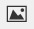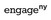PSA2QEK - Exit Tickets---Geometry, M1, Lesson 18 (G.CO.5)

Construct a line through the point P below that is parallel to the line l by rotating l by 180° (using the steps outlined in Example 2).

Steps outlined in Example 2:

a. Label any point A on l

b. Find the midpoint of segment AP using a ruler. (Measure the length of segment AP, and locate the point that is distance AP/2 from A between A and P.) Label the midpoint C.

c. Perform a 180° rotation around center C. To quickly find the image of l under this rotation by hand:

1. Pick another point B on l
2. Draw.
3. Draw circle: center C, radius CB.
4. Label the other point where the circle intersectsby Q.
5. Drawd. Label the image of the rotation by 180° of l by l' = Rc,180(l).

Draw your graph on paper, take a picture, and upload it using the image upload icon.

If you do not have the ability to upload an image of your work, type “Graph is on paper."Modified from EngageNY ©Great Minds Disclaimer